LIFEwork balance We have started a LIFEworkbalance campaign

• Slides: 22LIFE/work balance We have started a #LIFEworkbalance campaign and we need your help to complete our LIFE/work balance survey. We hope to publish the results soon, so please give 15 minutes of your time to help us get a true picture of school life. Want to be a part of this campaign? Take the survey on our website and share it with your colleagues! © Classroom Secrets Limited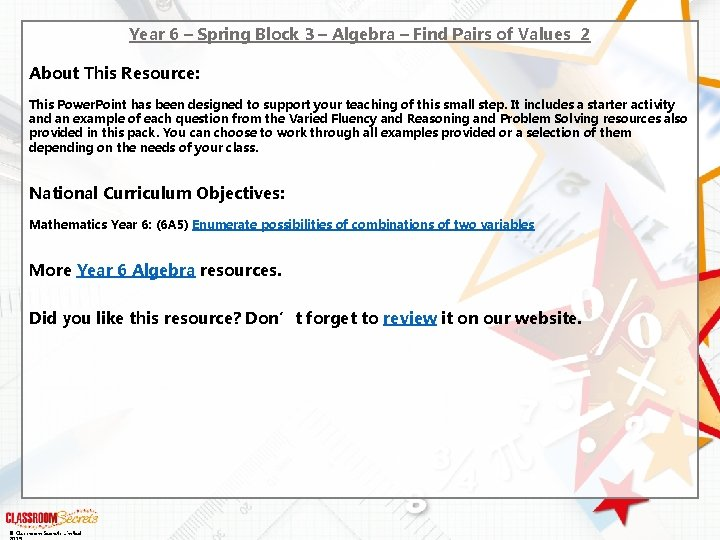Year 6 – Spring Block 3 – Algebra – Find Pairs of Values 2 About This Resource: This Power. Point has been designed to support your teaching of this small step. It includes a starter activity and an example of each question from the Varied Fluency and Reasoning and Problem Solving resources also provided in this pack. You can choose to work through all examples provided or a selection of them depending on the needs of your class. National Curriculum Objectives: Mathematics Year 6: (6 A 5) Enumerate possibilities of combinations of two variables More Year 6 Algebra resources. Did you like this resource? Don’t forget to review it on our website. © Classroom Secrets Limited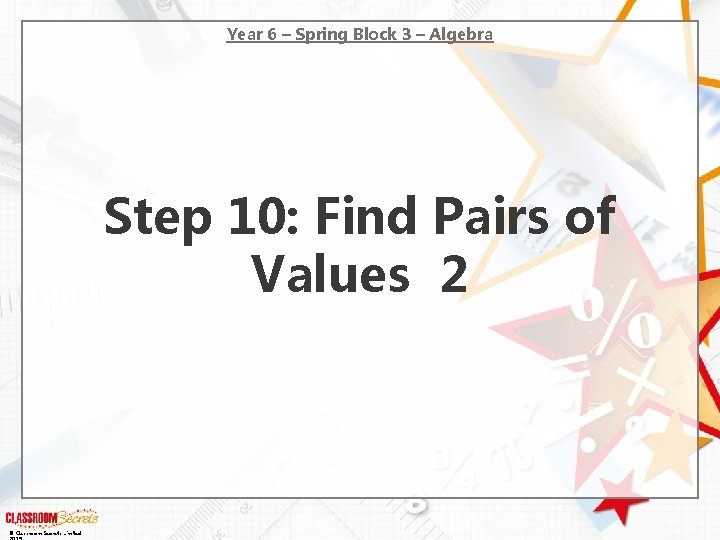Year 6 – Spring Block 3 – Algebra Step 10: Find Pairs of Values 2 © Classroom Secrets Limited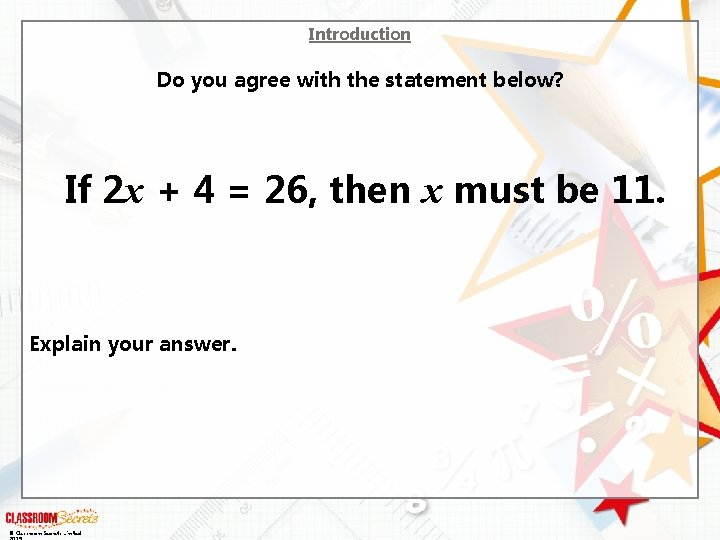Introduction Do you agree with the statement below? If 2 x + 4 = 26, then x must be 11. Explain your answer. © Classroom Secrets LimitedIntroduction Do you agree with the statement below? If 2 x + 4 = 26, then x must be 11. Explain your answer. I agree because… © Classroom Secrets Limited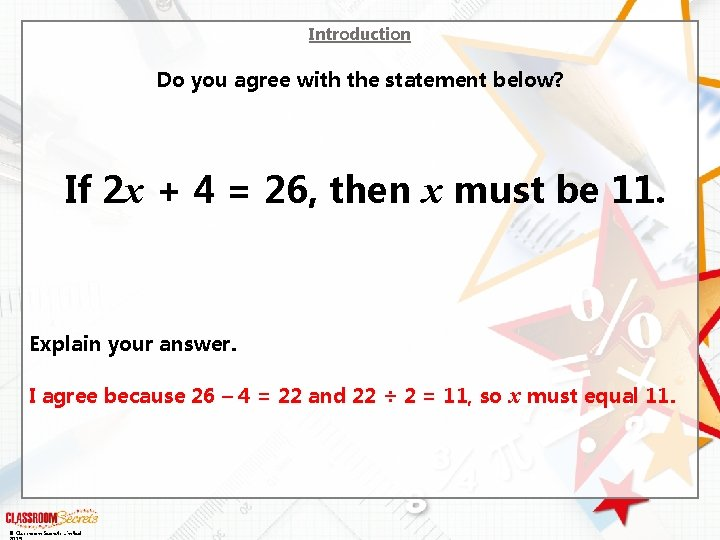Introduction Do you agree with the statement below? If 2 x + 4 = 26, then x must be 11. Explain your answer. I agree because 26 – 4 = 22 and 22 ÷ 2 = 11, so x must equal 11. © Classroom Secrets Limited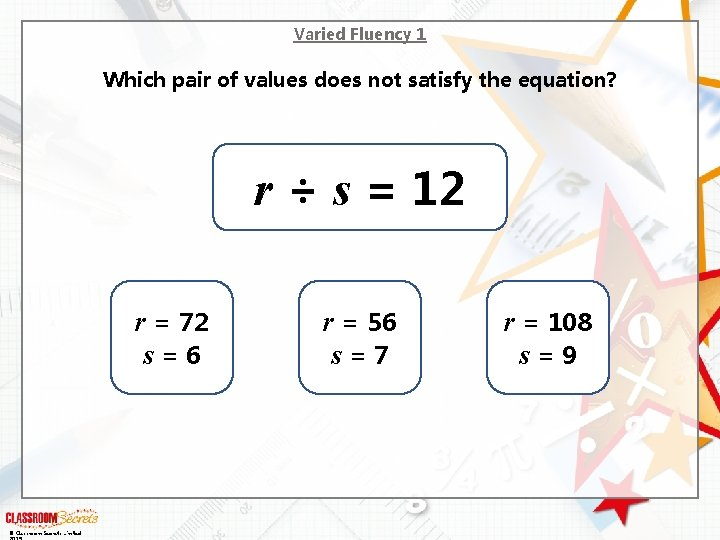Varied Fluency 1 Which pair of values does not satisfy the equation? r ÷ s = 12 r = 72 s = 6 © Classroom Secrets Limited r = 56 s = 7 r = 108 s = 9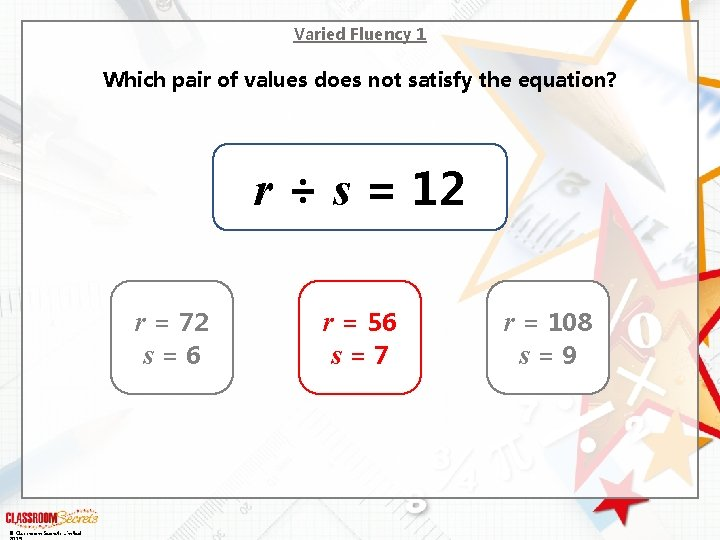Varied Fluency 1 Which pair of values does not satisfy the equation? r ÷ s = 12 r = 72 s = 6 © Classroom Secrets Limited r = 56 s = 7 r = 108 s = 9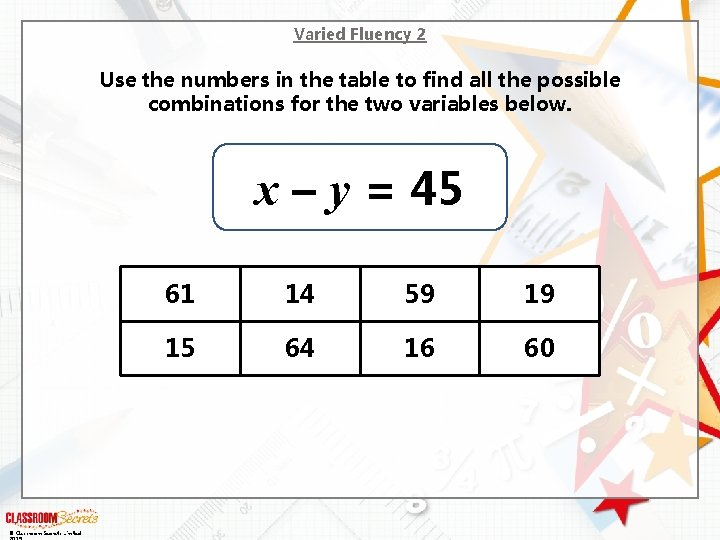Varied Fluency 2 Use the numbers in the table to find all the possible combinations for the two variables below. x – y = 45 © Classroom Secrets Limited 61 14 59 19 15 64 16 60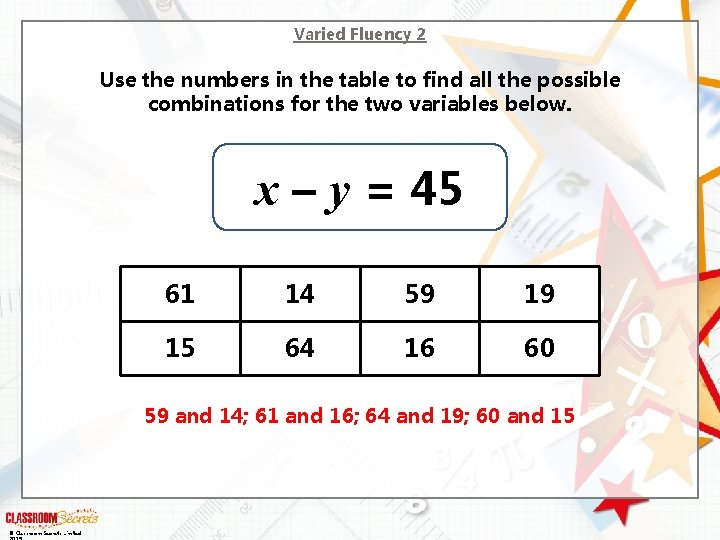Varied Fluency 2 Use the numbers in the table to find all the possible combinations for the two variables below. x – y = 45 61 14 59 19 15 64 16 60 59 and 14; 61 and 16; 64 and 19; 60 and 15 © Classroom Secrets LimitedVaried Fluency 3 Work out the values of b and c. a = 15 a + b = 25 c + b = 35 b = c = © Classroom Secrets LimitedVaried Fluency 3 Work out the values of b and c. a = 15 a + b = 25 c + b = 35 10 b = c = 25 © Classroom Secrets Limited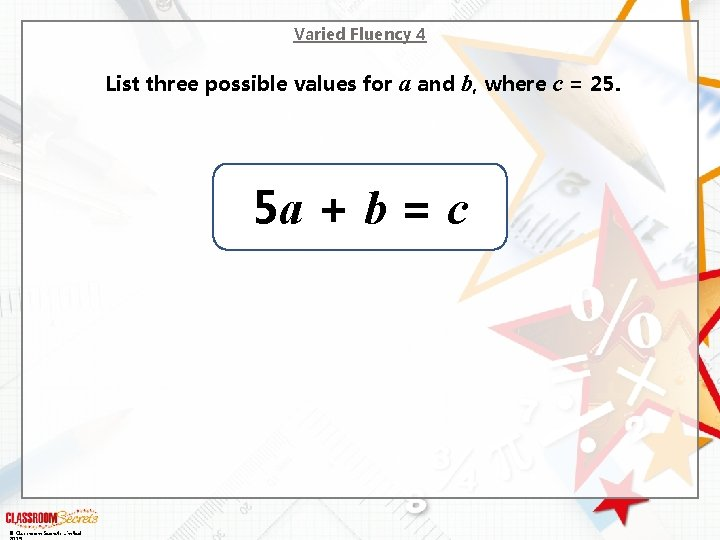Varied Fluency 4 List three possible values for a and b, where c = 25. 5 a + b = c © Classroom Secrets Limited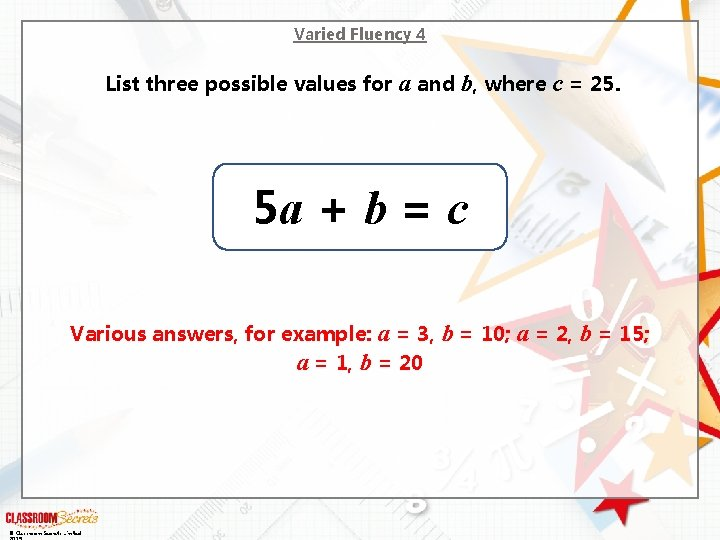Varied Fluency 4 List three possible values for a and b, where c = 25. 5 a + b = c Various answers, for example: a = 3, a = 1, b = 20 © Classroom Secrets Limited b = 10; a = 2, b = 15;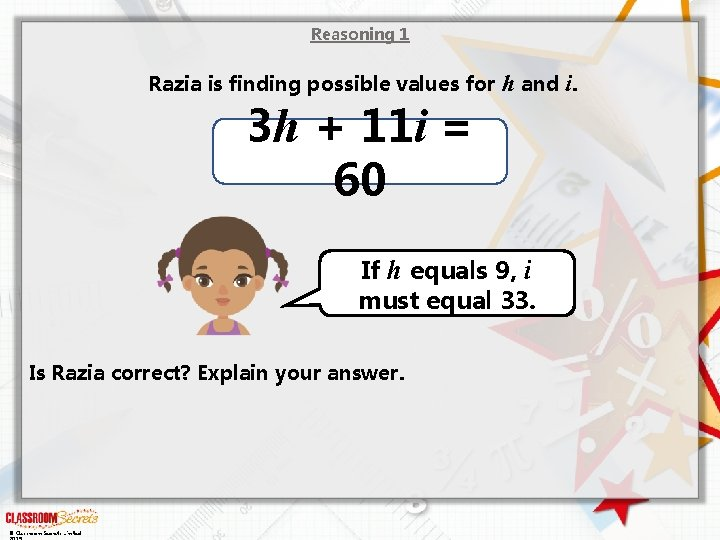Reasoning 1 Razia is finding possible values for h and i. 3 h + 11 i = 60 If h equals 9, i must equal 33. Is Razia correct? Explain your answer. © Classroom Secrets LimitedReasoning 1 Razia is finding possible values for h and i. 3 h + 11 i = 60 If h equals 9, i must equal 33. Is Razia correct? Explain your answer. Razia is incorrect because… © Classroom Secrets Limited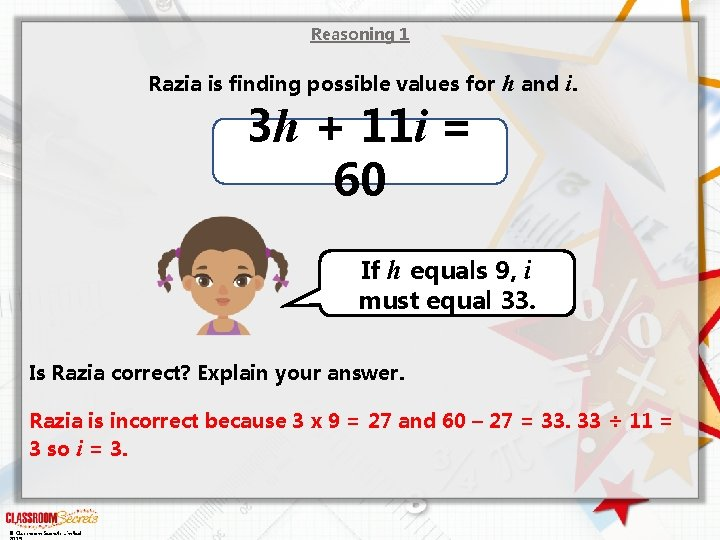Reasoning 1 Razia is finding possible values for h and i. 3 h + 11 i = 60 If h equals 9, i must equal 33. Is Razia correct? Explain your answer. Razia is incorrect because 3 x 9 = 27 and 60 – 27 = 33. 33 ÷ 11 = 3 so i = 3. © Classroom Secrets LimitedReasoning 2 If a is an odd number and b is 50, which of these could be true? A. 2 a + 3 b = 156 B. a + a – 3 b = 4 C. 4 a + 5 b = 258 D. 2 a ÷ 3 b = 2 Convince me. © Classroom Secrets LimitedReasoning 2 If a is an odd number and b is 50, which of these could be true? A. 2 a + 3 b = 156 B. a + a – 3 b = 4 C. 4 a + 5 b = 258 D. 2 a ÷ 3 b = 2 Convince me. A and B could be true because… © Classroom Secrets Limited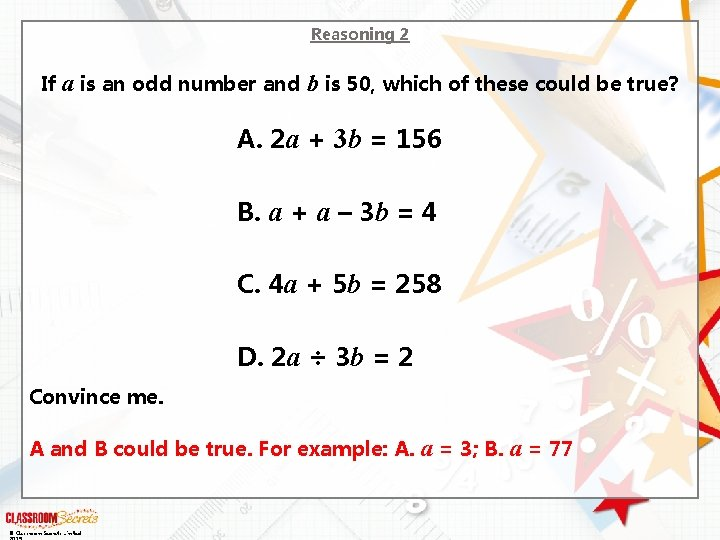Reasoning 2 If a is an odd number and b is 50, which of these could be true? A. 2 a + 3 b = 156 B. a + a – 3 b = 4 C. 4 a + 5 b = 258 D. 2 a ÷ 3 b = 2 Convince me. A and B could be true. For example: A. a = 3; B. a = 77 © Classroom Secrets LimitedProblem Solving 1 Sweety Treaty sell 2 medium sweet boxes and 4 small sweet boxes for £ 36. What possible prices can you find for each sweet box? 2 m + 4 s = £ 36 m © Classroom Secrets Limited s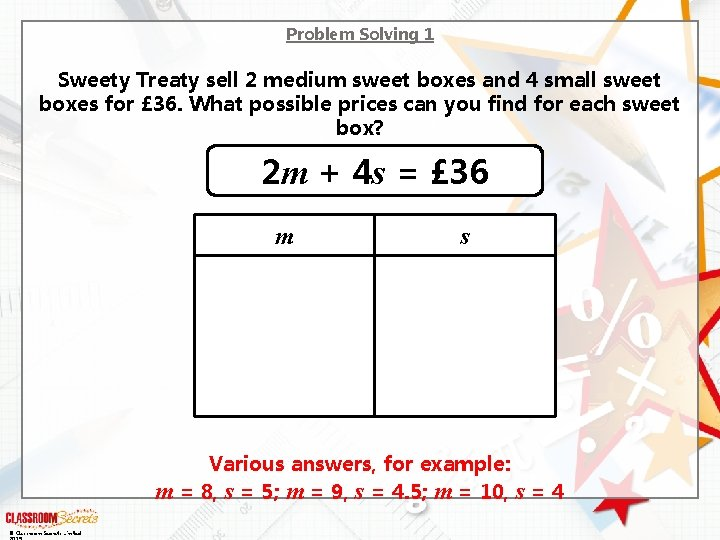Problem Solving 1 Sweety Treaty sell 2 medium sweet boxes and 4 small sweet boxes for £ 36. What possible prices can you find for each sweet box? 2 m + 4 s = £ 36 m s Various answers, for example: m = 8, s = 5; m = 9, s = 4. 5; m = 10, s = 4 © Classroom Secrets Limited